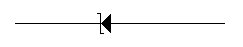# Electronics and Communication Engineering - Electronic Devices and Circuits

26.

The density of states (i.e. number of states per eV per m3) in the conduction band for energy level E is proportional to

 A. E B. E C. E1.5 D. E2

Answer: Option A

Explanation:

No answer description available for this question. Let us discuss.

27.

A-P type material has an acceptor ion concentration of 1 x 1016 per cm3. Its intrinsic carrier concentration is 1.48 x 1010/ cm. The hole and electron mobilities are 0.05m2/V-sec and 0.13 m2/V-sec respectively calculate the resistivity of the material

 A. 12.5 Ω-cm B. 1.25 Ω-cm C. 0.125 Ω-cm D. 125 Ω-cm

Answer: Option B

Explanation:

No answer description available for this question. Let us discuss.

28.

Resistivity is a property of a semiconductor that depends on

 A. the atomic weight of the semiconductor B. the atomic number of the semiconductor C. the atomic nature of the semiconductor D. the shape of the semiconductor

Answer: Option C

Explanation:

No answer description available for this question. Let us discuss.

29.

Operating point signifies that

 A. zero signal IC and VBE B. zero signal IC and VCE C. zero signal IB and VCE D. zero signal IC, VCE

Answer: Option B

Explanation:

No answer description available for this question. Let us discuss.

30.

Figure represents aA. Tunnel diode B. Zener diode C. Photo diode D. Photo sensitive diode

Answer: Option B

Explanation:

No answer description available for this question. Let us discuss.

#### Current Affairs 2021

Interview Questions and Answers